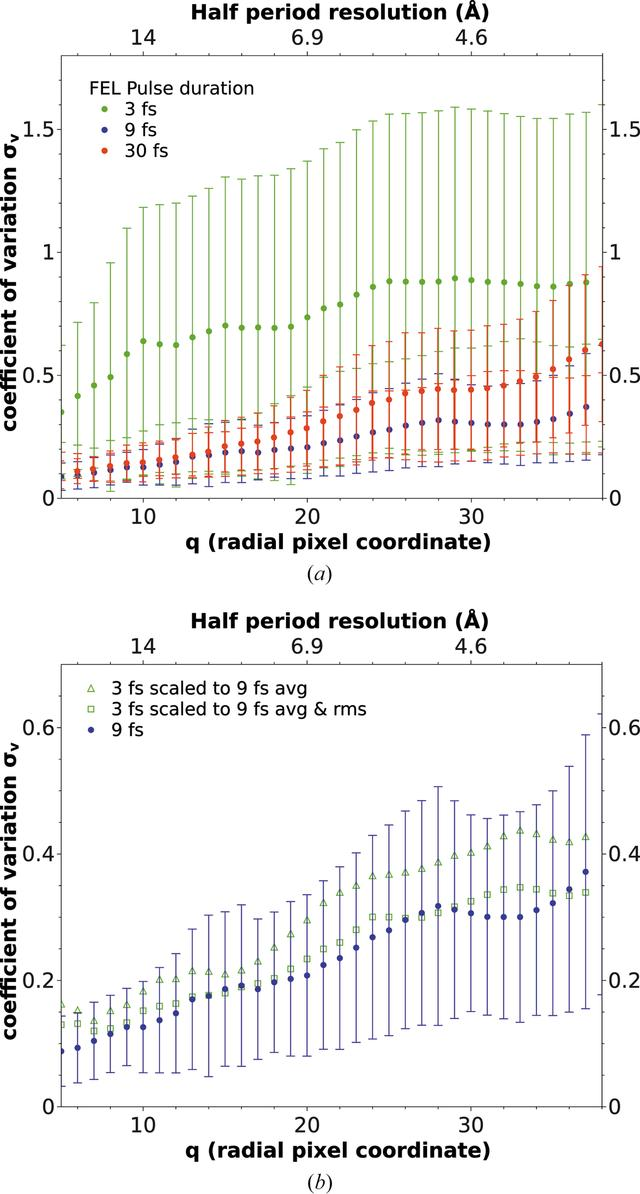disable zoom     view article Figure 3 (a) The coefficient of variation of oriented three-dimensional diffraction volumes for pulse durations of 3, 9 and 30 fs. (b) The coefficient of variation for a pulse duration of 9 fs and re-scaled coefficients for 3 fs. Triangles: every pattern has been multiplied by a constant factor of 3.3 to match the average photon count in the 9 fs patterns. Squares: every 3 fs pattern has been multiplied by an individual factor such that the average and r.m.s. photon counts match the 9 fs data.

IUCrJ
Volume 4| Part 5| September 2017| Pages 560-568
ISSN: 2052-2525